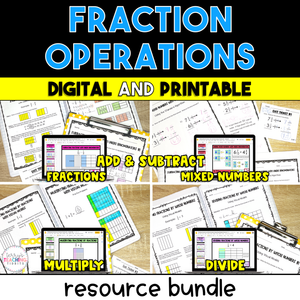# 5th Grade Fractions Bundle - Add, Subtract, Multiply, & Divide - Digital & Printable

• \$52.00
Unit price per
• Save \$18
Shipping calculated at checkout.

Use visual models along with the standard algorithm and area models to quickly and effectively teach your students how to add, subtract, multiply, and divide fractions and mixed numbers with this comprehensive printable & digital resource pack.

With 21 mini-lessons, (6 adding and subtracting fractions, 5 adding and subtracting mixed numbers, 6 multiplying fractions, and 4 dividing fractions) you will have plenty of resources to teach your student how to solve problems using fraction operations.

Using models, students will have a more hands-on approach to “see” what it looks like to add, subtract, multiply, and divide fractions.  These models will help reinforce the use and understanding of the standard algorithm.  It’s great for kinesthetic learners as well as students that may struggle with memorizing algorithms.

This resource is complete, comprehensive, and easy to follow with a clear format.  It is low-prep, easy to assign to a sub, and contains engaging printable activities and digital versions for use with Google Classroom.  If your students are struggling conceptually, this resource is for you!

Standards 5.NF.1, 5.NF.2, 5.NF.4, 5.NF.4a, 5.NF.4b, 5.NF.6, 5.NF.7, 5.NF.7a, 5.NF.7b, 5.NF.7c, 6.NS.1

This resource is also part of my 5th Grade Math Year-Long Curriculum Bundle.

How Can You Use This?

Introduce each skill with the detailed set of notes and sample problems.  I suggest doing this in a whole group setting and displaying the PDF or pulling the slides up on a Smartboard if you’re in person or share the screen online if you’re teaching virtually.

Gradually release students to practice independently using the practice pages or slides activities.

When all skills have been taught, provide additional practice and review using the set of printable or digital task cards (Add/Subt Fractions & Mixed numbers only), then assess with the final quizzes when you feel students are ready.

## What You Get

Mini-Lessons Included:

• Fraction Notes Pages for Finding the Least Common Multiple, Simplifying Fractions, and Converting Improper Fractions and Mixed Numbers
• Add Fractions with Like Denominators
• Subtract Fractions with Like Denominators
• Add Fractions with Unlike Denominators Using Visual Models
• Subtract Fractions with Unlike Denominators Using Visual Models
• Add Fractions with Unlike Denominators Using the Standard Algorithm
• Subtract Fractions with Unlike Denominators Using the Standard Algorithm
• Add Mixed Numbers with Like Denominators
• Subtract Mixed Numbers with Like Denominators
• Add Mixed Numbers with Unlike Denominators
• Subtract Mixed Numbers with Unlike Denominators (without Renaming)
• Subtract Mixed Numbers with Unlike Denominators (with Renaming)
• Multiply Fractions by Whole Numbers with Visual Models
• Multiply Fractions by Fractions with Visual Models
• Multiply Fractions by Whole Numbers and Fractions Using the Standard Algorithm
• Multiply Mixed Numbers with Area Models
• Multiply Mixed Numbers Using the Standard Algorithm
• Multiply Fractions Word Problems
• Divide Unit Fractions by Whole Numbers with Visual Models
• Divide Whole Numbers by Unit Fractions with Visual Models
• Divide Fractions Using the Standard Algorithm
• Divide Fractions with Word Problems

What You’ll Get in EACH of the mini-lessons:

• Detailed Notes
• Sample Problems
• Practice Pages
• Homework Pages (In select Multiplying Fractions Minilessons)
• Exit Tickets (2+ *The amount varies depending on the mini-lesson*)

You’ll Also Get:

• Quiz (assessment)